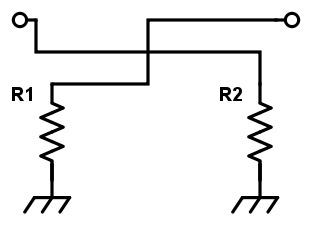# Reflection Attenuator Calculator

## This tool is designed to calculate the value of resistance R1 in a reflection attenuator.

(dB)
(Ω)

(Ω)
(Ω)

### Overview

This reflection attenuator calculator is designed to help in calculating the correct values of the resistor R1 according to the diagram below. The only requirement is the required attenuation in decibels (dB) and the impedance in ohms. Note that there are two formulas for R1: one where R1 is greater than the characteristic impedance of the line to be match and another where R1 is lesser than that characteristic impedance.### Equations

$$R_{1} = Z_{0}\left [ \frac{10^{\frac{A_{dB}}{20}}+1}{10^{\frac{A_{dB}}{20}}-1} \right ]$$             if $$R_{1} > Z_{0}$$

$$R_{1} = \frac{Z_{0}}{2}\left [ \frac{10^{\frac{A_{dB}}{20}}-1}{10^{\frac{A_{dB}}{20}}+1} \right ]$$             if $$R_{1} < Z_{0}$$

### Applications

Attenuators are passive circuits that are used to weaken the signal from a source to a level suitable for the destination. The source can be a transmitter circuit or a transmission line and the destination may be another transmission line or an antenna. Attenuators in RF applications are usually just a simple network of resistors.

Reflection attenuators have two or more discrete states (digital) and are most often they are used as variable phase shifters. Depending on the type of coupler used, such an attenuator can provide an octave bandwidth. In fact, reflection attenuators can provide invariant response with careful design.

Balanced Attenuator

Pi Attenuator Calculator

Bridged-Tee Attenuator Calculator